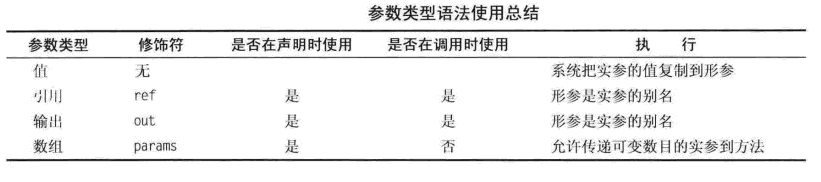C#方法中参数ref和out详解1、值参数

使用值参数，通过复制实参的值到形参的方式把数据传递到方法。方法调用时，系统做如下操作：

· 在栈中为形参分配空间

· 复制实参到形参

注：栈（先进后出）是编译期间就分配好的内存空间，因此你的代码中必须就栈的大小有明确的定义；

堆（队列优先,先进先出）是程序运行期间动态分配的内存空间，你可以根据程序的运行情况确定要分配的堆内存的大小。

```/// <summary>
/// 声明方法
/// </summary>
/// <param name="value">参数</param>
/// <returns>返回值</returns>
static float FuncData(float value )
{
float i = 1.5F;
float j = 2.5F;

float value1 = FuncData(i);    //方法调用
float value2 = FuncData(i+j);   //方法调用（一个值参数的实参不一定是变量。它可以是任何能计算成相应数据类型的表达式）
return value1 + value2;
}
```

2、引用参数

· 使用引用参数时，必须在方法的声明和调用中都使用ref修饰符

· 实参必须是变量，在用作实参前必须被赋值，如果是引用类型变量，可以赋值为一个引用或者null值

```/// <summary>
/// 声明方法
/// </summary>
/// <param name="value">参数</param>
/// <returns>返回值</returns>
static void FuncData(float value )
{
//方法调用
int temp=0;      //实参变量
FuncData(ref temp);  //包含修饰符ref
//FuncData(ref temp+1); //错误，必须使用变量
}

/// <summary>
/// 方法声明
/// </summary>
/// <param name="value">参数</param>
/// <returns>返回值</returns>
static int FuncData(ref int value)
{
return value = 100;
}

```

3、输出参数

· 必须在声明和调用中都使用修饰符。输出参数的修饰符是out不是ref

· 和引用参数相似，实参必须是变量，而不能是其他类型的表达式。（因为方法需要内存位置保存返回值）

```/// <summary>
/// 声明方法
/// </summary>
/// <param name="value">参数</param>
/// <returns>返回值</returns>
static void FuncData(float value )
{
//方法调用
int temp=0;      //实参变量
FuncData(out temp);  //包含修饰符out
//FuncData(out temp+1); //错误，必须使用变量
}

/// <summary>
/// 方法声明
/// </summary>
/// <param name="value">参数</param>
/// <returns>返回值</returns>
static int FuncData(out int value)
{
return value = 100;
}

```

· 在方法内部，输出参数在被读取之前必须被赋值 （意味着参数的初始值是无关的，而且没有必要在方法调用之前为实参赋值）

· 在方法返回之前，方法内部的任何贯穿的可能路径都必须为所有输出参数进行一次赋值。

```class MyClass {
public int val = 100;
}

class Program
{
/// <summary>
/// 方法声明
/// </summary>
static void FuncData(out MyClass myclass,out int temp)
{
myclass = new MyClass(); //创建一个类变量
myclass.val = 50;     //赋值字段
temp=20;         //赋值int参数
}

/// <summary>
/// 方法调用
/// </summary>
static void Main()
{
MyClass my = null;
int temp;
FuncData(out my,out temp);//调用方法
}

static void FuncTest(out int value)
{
var test = value+1;//错误，在方法赋值之前无法读取输出变量
}
}

```

4、参数数组

·在一个参数列表中只能有一个参数数组

·如果有，它必须是列表中的最后一个

声明一个参数数组必须做的事

·在数据类型前使用Params修饰符

·在数据类型后放置一组空的方括号

```/// <summary>
/// 声明方法
/// </summary>
/// <param name="Array">参数</param>
static void FuncTest(params int[] Array)
{
...
}
```

· 数组是一组整齐的相同类型的数据项

· 数组使用一个数字索引进行访问

· 数组是一个引用类型，因此它的所有数据项都保存在堆中

1、使用ref型参数时，传入的参数必须先被初始化。对out而言，必须在方法中对其完成初始化

2、使用ref和out时，在方法的参数和执行方法时，都要加Ref或Out关键字，以满足匹配。

3、out适合用在需要retrun多个返回值的地方，而ref则用在需要被调用的方法修改调用者的引用的时候。

```class TestApp
{
static void outTest(out int x, out int y)
{
//离函数前必须xy赋值否则报错
//y = x;
//上面行报错使用outxy都清空需要重新赋值即使调用函数前赋值行
x = 1;
y = 2;
}

static void refTest(ref int x, ref int y)
{
x = 1;
y = x;
}

public static void Main()
{
//正确 （out test）
int a, b;
//out使用前变量赋值
outTest(out a, out b);
Console.WriteLine("a={0};b={1}", a, b);
//在使用out关键字时，不需要在此处初始化，初始化也不会影响到方法内部的值，所以你初始化没用
int c = 11, d = 22;
outTest(out c, out d);
Console.WriteLine("c={0};d={1}", c, d);

//错误 （ref test）
int m, n;
refTest(ref m, ref n);
//上面行错ref使用前变量必须赋值

//正确（ref test）
int o = 11, p = 22;
refTest(ref o, ref p);
Console.WriteLine("o={0};p={1}", o, p);
}
}

```

1、ref的使用：使用ref进行参数的传递时，该参数在创建时，必须设置其初始值，且ref侧重于修改；

2、out的使用：采用out参数传递时，该参数在创建时，可以不设置初始值，但是在方法中必须初始化，out侧重于输出；

注释：当希望方法返回多个值时，可以用out，并且一个方法中的参数可以有一个或多个out参数；使用out参数，必须将参数作为out参数显式传递到方法中，但是out 参数的值不会被传递到 方法中，且属性不是变量，不能作为 out 参数传递。

ref是有进有出，而out是只出不进。